# # 3D数学基础

## # 向量

### # 向量大小

``````Vector3 v1 = new Vector3(1, 2, 3);
//长度
Debug.Log(v1.magnitude);
//长度的平方，可节省计算性能
Debug.Log(v1.sqrMagnitude);
``````
1
2
3
4
5

### # 向量方向

``````Vector3 v1 = new Vector3(1, 2, 3);
Debug.Log(v1.normalized);//返回标准化的结果
Debug.Log(v1);
v1.Normalize();//将v1自身标准化
Debug.Log(v1);
``````
1
2
3
4
5

### # 三角函数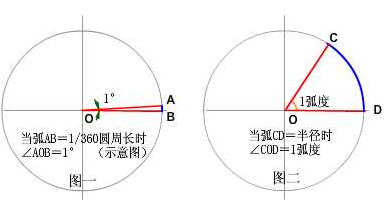PI=180度，1弧度=180度/PI，1角度=PI/180度

Unity3d中常用的三角函数运算：

``````弧度=角度数*Mathf.Deg2Rad

``````
1
2
3
4
5
6
7
8
9
10

### # 向量点乘

a·b=|a| · |b| cos/<a,b/>

``````float dot =Vector3.Dot(a.normalized, b.normalized);
float angle = Mathf.Acos(dot) * Mathf.Rad2Deg;
``````
1
2

### # 向量叉乘

[x1,y1,z1] x [x2,y2,z2] = [y1 * z2 - z1 * y2, z1 * x2 - x1 * z2, x1 * y2 - y1 * x2] a·b=|a| · |b| sin/<a,b/>

``````Vector3 cross = Vector3.Cross(a.normalized, b.normalized);
float angle = Mathf.Asin(cross.magnitude) * Mathf.Rad2Deg;
``````
1
2

### # 常用API

``````Vector3.Lerp
Vector3.MoveTowards
Vector3.SmoothDamp
Vector3.Angle
Vector3.ProjectOnPlane
Vector3.Reflect
``````
1
2
3
4
5
6

## # 欧拉角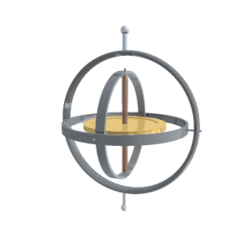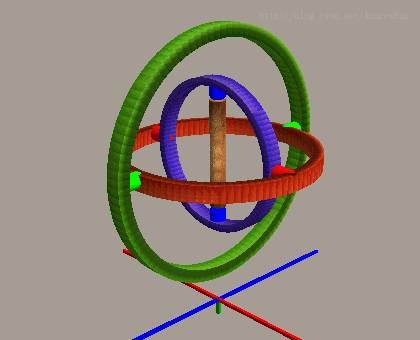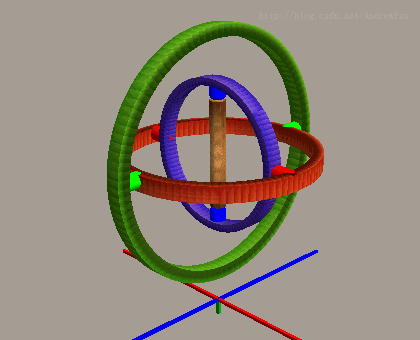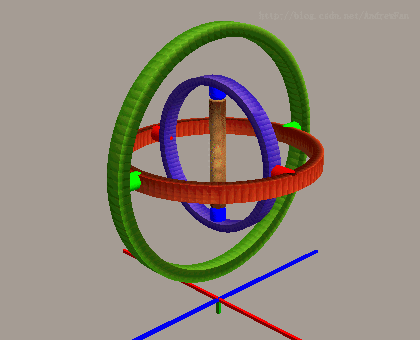• 仅使用三个数字表达方位，占用空间小。
• 沿坐标轴旋转的单位为角度，符合人的思考方式。
• 任意三个数字都是合法的，不存在不合法的欧拉角。

Vector3 eulerAngle = this.transform.eulerAngles;

### # 万向节死锁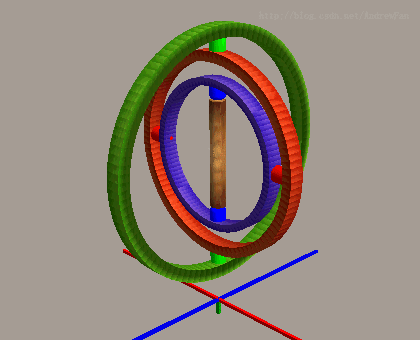## # 四元数

Quaternion 在3D图形学中代表旋转，由一个三维向量(X,Y,Z)和一个标量(W)组成。

• x=sin(θ /2)*V.x
• y=sin(θ /2)*V.y
• z=sin(θ /2)*V.z
• w=cos(θ /2)

X、Y、Z、W的取值范围是-1到1。Unity3d中获取四元数：

Quaternion qt = this.transform.rotation;

### # 四元数运算

``````Vector3 point = new Vector3(0,0,10);
Vector3 newPoint = Quaternion.Euler(0,30,0) * point ;
``````
1
2

``````Quaternion rotation01 = Quaternion.Euler(0, 30, 0) * Quaternion.Euler(0, 20, 0);
Quaternion rotation02 = Quaternion.Euler(0, 50, 0);
``````
1
2

``````this.transform.rotation *= Quaternion.Euler(0, 1, 0); //可使物体沿自身坐标Y轴旋转
this.transform.Rotate(Vector3 eulerAngles) //内部就是使用四元数相乘实现
``````
1
2

### # 常用接口

``````Quaternion.LookRotation
Quaternion.Euler
Quaternion.Lerp
Quaternion.FromToRotation
Quaternion.AngleAxis
``````
1
2
3
4
5